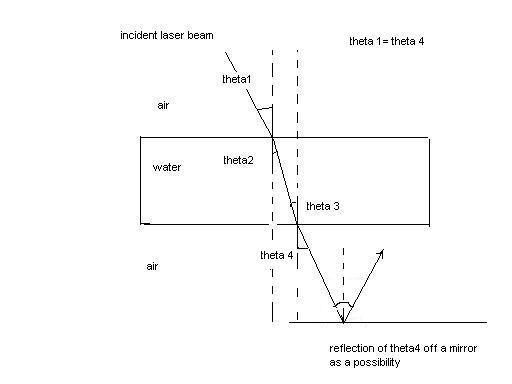# How does one measure the index of refraction of a liquid, and . . . ?

How does one measure the index of refraction of a liquid, more specifically the angle of refraction? I'm finding it's relationship to temperature.I can't just dip the protractor into the liquid( as a test medium I used water compared to air) and measure the angle that is in the water(either theta 2 or theta 3). Moreover, the incident angle theta 1 is congruent to the outgoing angle theta 4.

Also the water is placed in a plastic container with rectangular sides to minimize curvature. I'm not sure if the plastic will affect the overall index of refraction/refraction angle in the water itself or not. The plastic is transparent and very thin. Glass containers may be used later.
I'm just looking for any ideas on how to measure the refraction angle without actually measuring it through the water. One possibility or hypothesis is to reflect the angle outgoing(theta 4) off a mirror and then measure the change in refraction somehow in that way. As the temperature changes(- or + direction) if the refraction angle(theta 2 or 3) becomes smaller the distance opposite and the hypotenuse of the angle(ie ratio to find sine theta) becomes smaller which might lead in the change of the angle theta 4 .

Integral
Staff Emeritus
$$\theta = Tan^{-1} \frac d h$$
$$\theta = \frac d h$$